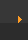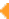## Chemical Bonding: Lewis Dot Structure for C2H4 (6 of 6)

Watch the video of Dr. B. drawing the Lewis dot structure for C2H4 (ethene) and answer the questions below.

Note that the C2H4 Lewis dot structure involves sharing more than one pair of electrons.

Hint: look at the questions before watching the video.

Video Transcript: OK, we're going to do one here, this is called ethene. It's C2H4, and we want to write the dot structures for ethene. To do that, we always count our valence electrons up first. Let's take a look: Carbon is in group 4, sometimes written 14, so it has 4 valence electrons. If we come way over here to Hydrogen, it's in group 1: it has 1 valence electron. So 4 for the Carbon, but there's two of them so we multiply it times 2. Plus one for the Hydrogen, but there's four of those so we multiply that times 4. So 2 tiems 4 is 8, plus 4 is 12. We have a total of 12 valence electrons here.

We're going to have to spread them around. Let's just write out--we always put the Hydrogens (let's move down some here) on the outside. So let's put our 4 Hydrogens on the outside, and let's ignore this right here. Let's put 2 electrons between each bond, so they're chemically bonded, lower energy, more stable, and then one in the middle. So I've used 2, 4, 6, 8, 10, I've got 2 more, so I'll put those out here. But my problems is that I've got 2, 4, 6, 8 for that one, but this Carbon only has 6. So I can't, with those 12 electrons, satisfy the octet. So what I'm going to do is take these and stick them right here in what's called a double bond.

Now if I cover up this side, I've got 2, 4, 6, 8. If I cover up this side, I've got 2, 4, 6, 8 and I've only used 2, 4, 6, 8, 10, 12. So I used my 12 electrons to form a double bond here that gives Carbon an octet; and then Hydrogen, they each have 2, so their outer shells are full. Sometimes you'll see that written like this: the double bond showing you the two pairs. I suppose you could write your H's; often they'll show the single bond like that with the H's. That's kind of an abbreviated format for the dots. So there you have it: ethene.